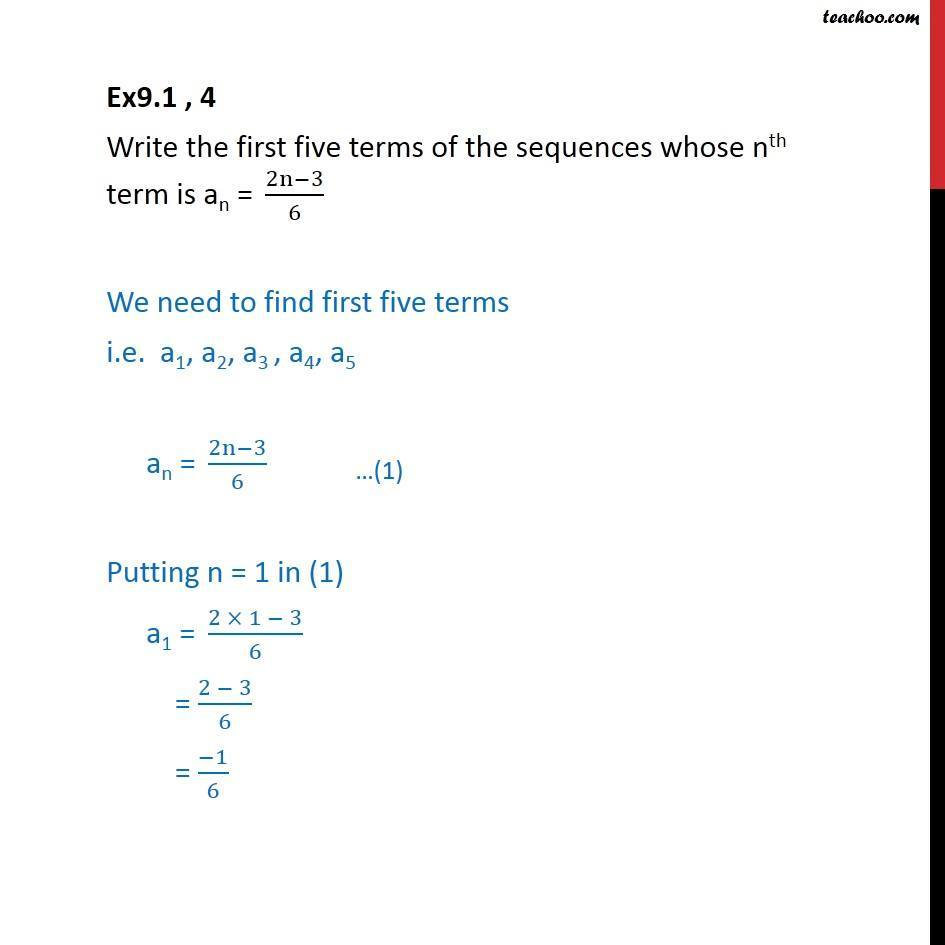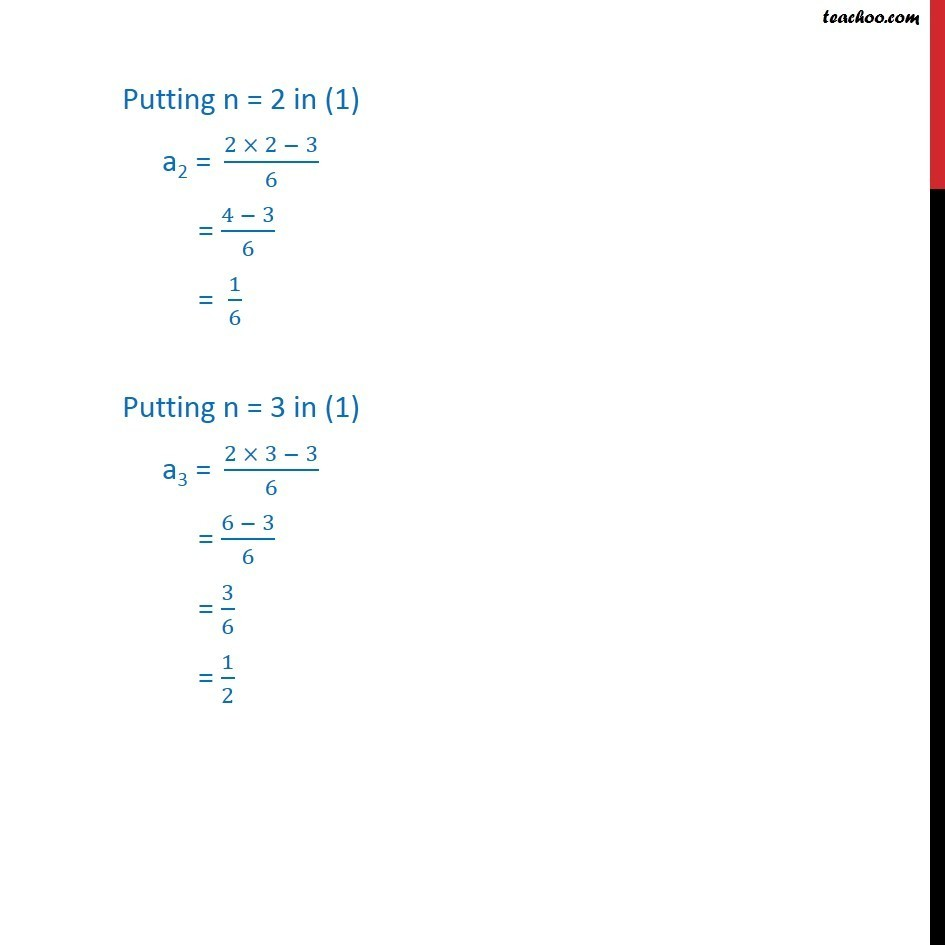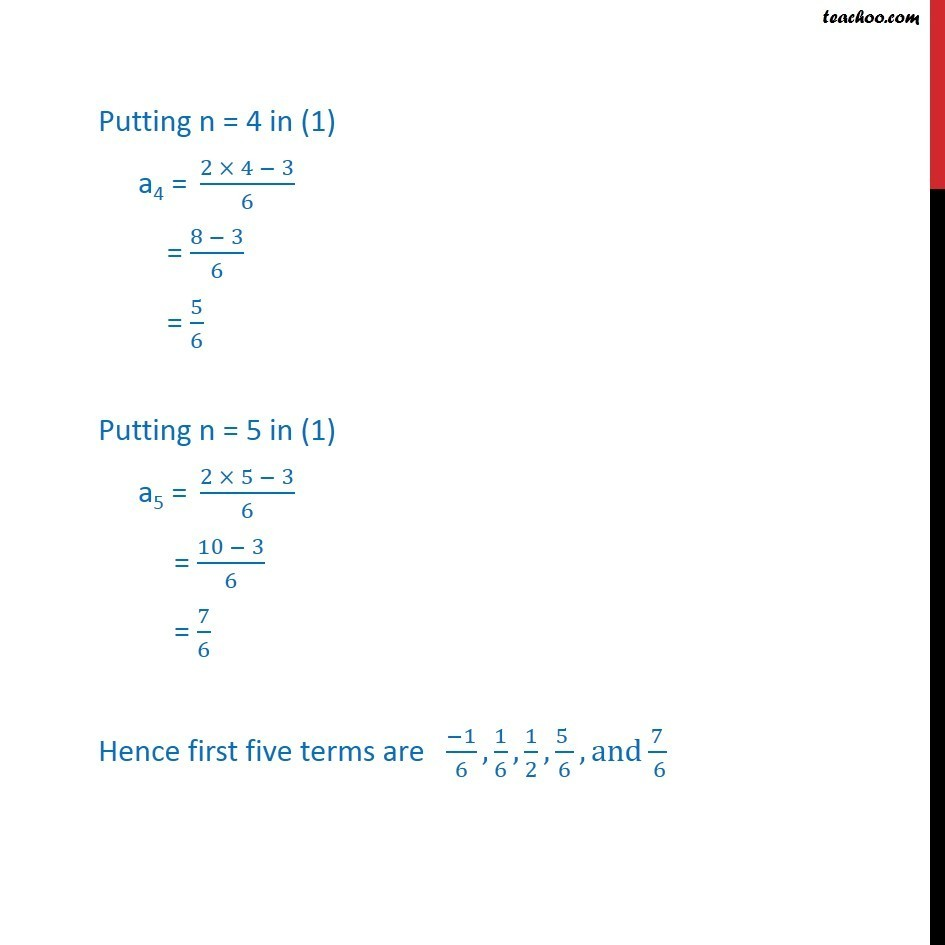Ex 8.1

Chapter 8 Class 11 Sequences and Series
Serial order wiseLearn in your speed, with individual attention - Teachoo Maths 1-on-1 Class

### Transcript

Ex9.1 , 4 Write the first five terms of the sequences whose nth term is an =" " (2n−3)/6 We need to find first five terms i.e. a1, a2, a3 , a4, a5 an =" " (2n−3)/6 Putting n = 1 in (1) a1 =" " (2 × 1 − 3)/6 = (2 − 3)/6 = (−1)/6 Putting n = 2 in (1) a2 =" " (2 × 2 − 3)/6 = (4 − 3)/6 = 1/6 Putting n = 3 in (1) a3 =" " (2 × 3 − 3)/6 = (6 − 3)/6 = 3/6 = 1/2 Putting n = 4 in (1) a4 =" " (2 × 4 − 3)/6 = (8 − 3)/6 = 5/6 Putting n = 5 in (1) a5 =" " (2 × 5 − 3)/6 = (10 − 3)/6 = 7/6 Hence first five terms are (−1)/6, 1/6, 1/2, (5 )/6, and 7/( 6)